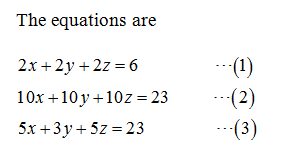# Solve. (Enter your answers as a comma-separated list. If there are infinitely many solutions, enter a parametric solution. If there is no solution, enter NONE.)2x + 2y + 2z = 610x + 10y + 10z = 235x + 3y + 5z = 23(x, y, z) =   )

Question
1 views

Solve. (Enter your answers as a comma-separated list. If there are infinitely many solutions, enter a parametric solution. If there is no solution, enter NONE.)

 2x + 2y + 2z = 6 10x + 10y + 10z = 23 5x + 3y + 5z = 23

(x, y, z) =

)

check_circle

Given:...

### Want to see the full answer?

See Solution

#### Want to see this answer and more?

Solutions are written by subject experts who are available 24/7. Questions are typically answered within 1 hour.*

See Solution
*Response times may vary by subject and question.
Tagged in